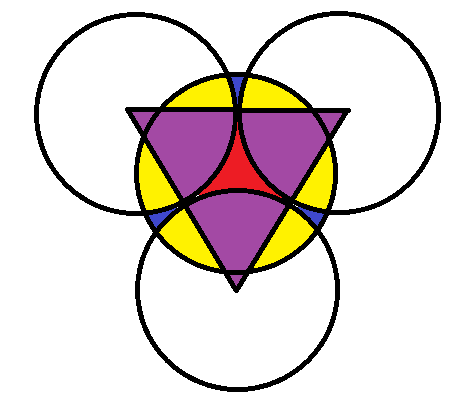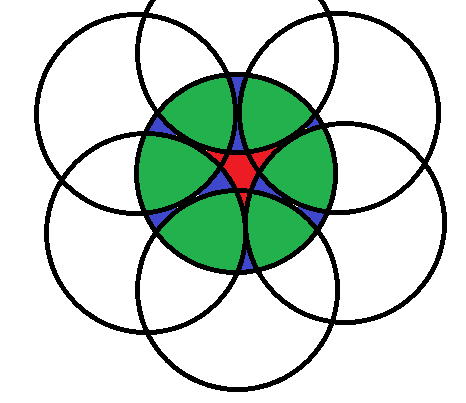BrainDen.com - Brain Teasers

Question

Let's say that there are three congruent circles that are tangent to each other. Draw a fourth congruent circle with its center at the center of the gap formed between the three circles. If all four circles are unit circles, what is the area of the overlap?

Recommended Posts

• 0
On 8/17/2017 at 0:42 AM, araver said:

Not sure what "really nice" means.

Hide contents

A nicer version would be to compute the area of the rhomboid and subtract it from twice the area of the sectors to obtain the yellow area.

The rhomboid has all lengths 1 and one diagonal equal to the green line above 2/sqrt(3) which makes the other diagonal 2*sqrt(2)/sqrt(3). The area of the rhomboid is then 2*sqrt(2)/3.

However computing the sector of the circle requires knowing the angle. Cosine law gives us cos(alpha) = -1/3 or using the rhomboid area above and the sine formula for an isosceless triangle sin(alpha) = 2*sqrt(2)/3. Area of one sector of the circle is alpha/2 = arcsin(2*sqrt(2)/3)/2.

Hence area of 1 yellow zone is alpha (2 sectors) - 2*sqrt(2)/3 (rhomboid).

Area of the required 3 yellow zones is then 3*arcsin(2*sqrt(2)/3) - 2*sqrt(2).

Pretty sure arccos(-1/3) is very irrational.

Since the above was never translated to an approximation (8 significant digits):

Spoiler

Area of the 3 yellow zones per above spoiler:

3*arcsin(2*sqrt(2)/3) - 2*sqrt(2) = 3*arccos(-1/3)-2*sqrt(2)=3*1.91063324-2*1.414213562 = 2.903472595

P.S. Area of the red sector per previous spoiler:

sqrt(3) - pi/2 = 1.732050808 - 3.141592654/2 = 0.161254481

Share on other sites

• 0

This is a nice one. I really think it should have a nice geometrical solution, but:

Spoiler

Dividing the area of the overlap means we should compute the area of the 3 equal intersections marked with yellow.

It would be easier to compute if we had the red area and the blue area, then subtract it from an unit circle's area.The red area is easier if we consider the purple triangle formed by the centers of the initial 3 circles.
The purple area plus the red area equals the area of an equilateral triangle of length 2 (since circles have a unit radius aka "unit circles"). The 3 purple areas are 60 degrees sectors each, hence the sum of the 3 areas is half a circle so pi/2. The triangle area is sqrt(3)*2^2/4. So the red area is sqrt(3) - pi/2.The blue areas are harder to findTrying to draw other 3 circles and recompute the areas otherwise only turns the problem into finding green areas which represent intersections between 3 circles this time.So, back to the drawing board, trying to compute the yellow area as a regular 2-circle intersection:

The length of the green segment is the hypotenuse of a rectangular triangle where an edge equal to 1 corresponds to a 60 degrees angle, hence green = 2/sqrt(3).

The length of the blue segment is equal to half the length of the radius (1) minus the part that exceeds the triangle (purple). The purple is (2/sqrt(3)-1)/2 = 1/sqrt(3)-1/2 (aprox .078), hence the blue length is h = 3/4-sqrt(3)/6 (aprox .46). This is the length of the height of the sector.

Half on a yellow area is given by the area of the sector minus the area of the isosceless triangle as cos-1(1-h) - (1-h) sqrt(2*h-h^2) which leads to the not nice formula cos-1((3+2*sqrt(3))/12) - (1/4+sqrt(3)/6)*sqrt((123-12*sqrt(3))/12). Multiplied by 6 to give the original asked area. Not sure if it can be reduced further to a nicer formula.

Share on other sites

• 0
27 minutes ago, araver said:

This is a nice one. I really think it should have a nice geometrical solution, but:

Hide contents

Dividing the area of the overlap means we should compute the area of the 3 equal intersections marked with yellow.

It would be easier to compute if we had the red area and the blue area, then subtract it from an unit circle's area.

The red area is easier if we consider the purple triangle formed by the centers of the initial 3 circles.
The purple area plus the red area equals the area of an equilateral triangle of length 2 (since circles have a unit radius aka "unit circles"). The 3 purple areas are 60 degrees sectors each, hence the sum of the 3 areas is half a circle so pi/2. The triangle area is sqrt(3)*2^2/4. So the red area is sqrt(3) - pi/2.

The blue areas are harder to findTrying to draw other 3 circles and recompute the areas otherwise only turns the problem into finding green areas which represent intersections between 3 circles this time.

So, back to the drawing board, trying to compute the yellow area as a regular 2-circle intersection:

The length of the green segment is the hypotenuse of a rectangular triangle where an edge equal to 1 corresponds to a 60 degrees angle, hence green = 2/sqrt(3).

The length of the blue segment is equal to half the length of the radius (1) minus the part that exceeds the triangle (purple). The purple is (2/sqrt(3)-1)/2 = 1/sqrt(3)-1/2 (aprox .078), hence the blue length is h = 3/4-sqrt(3)/6 (aprox .46). This is the length of the height of the sector.

Half on a yellow area is given by the area of the sector minus the area of the isosceless triangle as cos-1(1-h) - (1-h) sqrt(2*h-h^2) which leads to the not nice formula cos-1((3+2*sqrt(3))/12) - (1/4+sqrt(3)/6)*sqrt((123-12*sqrt(3))/12). Multiplied by 6 to give the original asked area. Not sure if it can be reduced further to a nicer formula.

Your solution does simplify to something really nice.  I will see if anyone sees it before I give it away.  Nice work.

Share on other sites

• 0

Your solution does simplify to something really nice.  I will see if anyone sees it before I give it away.  Nice work.

Not sure what "really nice" means.

Spoiler

A nicer version would be to compute the area of the rhomboid and subtract it from twice the area of the sectors to obtain the yellow area.

The rhomboid has all lengths 1 and one diagonal equal to the green line above 2/sqrt(3) which makes the other diagonal 2*sqrt(2)/sqrt(3). The area of the rhomboid is then 2*sqrt(2)/3.

However computing the sector of the circle requires knowing the angle. Cosine law gives us cos(alpha) = -1/3 or using the rhomboid area above and the sine formula for an isosceless triangle sin(alpha) = 2*sqrt(2)/3. Area of one sector of the circle is alpha/2 = arcsin(2*sqrt(2)/3)/2.

Hence area of 1 yellow zone is alpha (2 sectors) - 2*sqrt(2)/3 (rhomboid).

Area of the required 3 yellow zones is then 3*arcsin(2*sqrt(2)/3) - 2*sqrt(2).

Pretty sure arccos(-1/3) is very irrational.

Share on other sites

• 0

? arccos(-1/3) = 109.5 degrees.

I believe that is an approximation.

It is irrational because every trigonometric (and inverse) is irrational at a non-zero rational point. What I meant was "not nice" as in "not nice irrational" i.e. even arccos(-1/3)/pi is irrational.

Share on other sites

• 0
Spoiler

1. I constructed three tangent circles

2. Used the tangent locations to construct the center  and thus the location of the fourth circle calling this circle C

3. Found where the center circle overlapped with one of the original three, calling this circle A (arbitrarily chose a circle that comes from 'above' at the point of intersection with my center circle)

4. Integrated the upper portion of Circle A between the two points of intersection

5. Integrated the lower portion of circle c between the two points of intersection

6. Subtracted the two areas to derive the area of their overlap.

7. Multiplied that amount by 3 to derive the overall area.

Share on other sites

• 0

Red area:  alternate (and harder) methodSpoiler

Wolfram gives the filling factor of hexagonal circle packing as f = [pi sqrt(3)]/6 = 0.9068996821.

Spoiler

(A circle plus two red areas) tessellates the plane.
The circles have unit radii and pi areas.

f(circle + 2 red) = circle
f(pi + 2 red) = pi.

red = pi (1-f)/2f = 0.1612544807739810

Share on other sites

• 0
Spoiler

I did the same thing araver did the second time, and found the fraction of the circle subtracted by the triangle. For this, I only needed to look at the intersection between two of the circles.

First, I found the triangle made by the circle's center and the two points of intersection. This triangle has side lengths of 1, 1, and 2sqrt(2/3). Next, the area of this circle is sqrt(2)/3. Then, I solved for the angle connecting to the center, which is sec(-1/3). Now, I can use that to find the fraction of the circle, which is sec(-1/3)*pi/360. Subtracting that by sqrt(2)/3 gets us half of the intersection, which is about 0.48. Multiply that by 6 to get the area of the whole yellow section, which is approximately 2.90347.

Join the conversation

You can post now and register later. If you have an account, sign in now to post with your account.×   Pasted as rich text.   Paste as plain text instead

Only 75 emoji are allowed.

×   Your previous content has been restored.   Clear editor

×   You cannot paste images directly. Upload or insert images from URL.